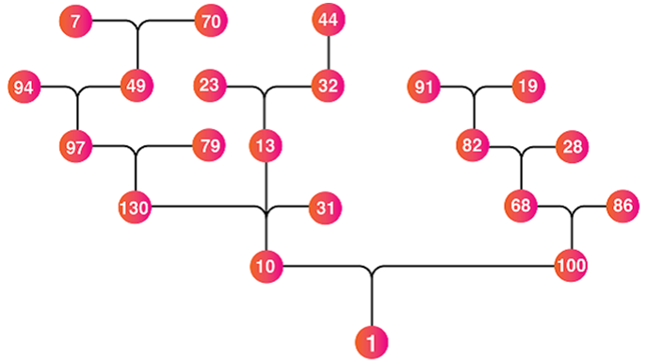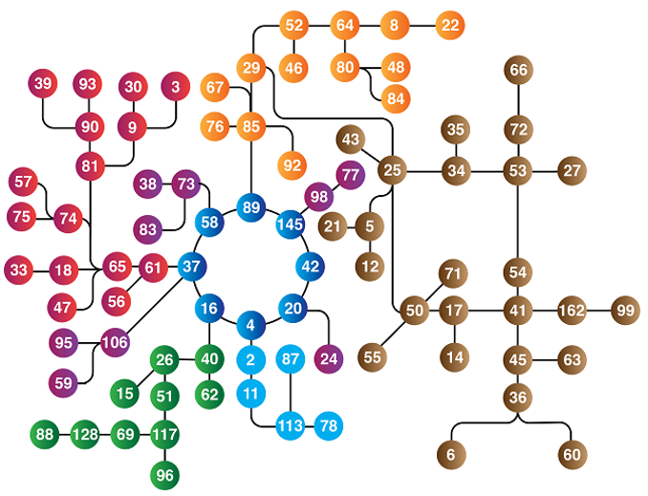## Want to keep learning?

This content is taken from the National STEM Learning Centre's online course, Maths Subject Knowledge: Understanding Numbers. Join the course to learn more.
4.11

# Happy numbers

As with perfect numbers, there is no practical application for happy numbers. This activity to create sequences of numbers to discover whether a number is a “Happy” number, requires students to follow a simple algorithm, produce unfamiliar sequences and practise squaring numbers.

To find if a number is happy:

• Replace the number by the sum of the squares of its digits.
• Repeat the process until either
• the next number in the sequence is 1, or
• the sequence loops endlessly in a cycle that does not include 1.

When the sequence gets to 1 then this is the end of the sequence as all subsequent terms in the sequence would be 1. All the numbers in this sequence are happy numbers, otherwise, it is an unhappy number.

Starting with 23 as an example:

$$2^2 = 4$$
$$3^2 = 9$$
$$4 + 9 = 13$$

Hence 13 is the next number in the sequence.

The process is now repeated:

$$1^2 = 1$$
$$3^2 = 9$$
$$1 + 9 = 10$$

Hence 10 is the next number in the sequence.

The process is now repeated:

$$1^2 = 1$$
$$0^2 = 0$$
$$1 + 0 = 1$$

Hence 1 is the next number in the sequence.

The sequence ends at this point since $$1^2 = 1$$.

From this we conclude that 23, 13, 10 and 1 are happy numbers.

Not all sequences lead to 1 and a sequence of happy numbers. A sequence of unhappy numbers migrate towards a cyclical loop that includes 4, 16, 37, 58, 89, 145, 42, and 20

Here is an interconnected chain of happy numbers, all leading downwards to one. It does not contain all possible happy numbers.When considering numbers between 1 and 100, some of the paths for unhappy numbers can be quite lengthy, for example, 60 takes nine intermediate steps before migrating to the cyclical loop.

The image below illustrates the connections between unhappy numbers.## Problem worksheet

Now complete question 12 from this week’s worksheet

You can read more about happy numbers on the STEM Learning website.

This is the last step of maths content for this course. The next step is a test, which is available if you upgrade and wish to get recognition of your understanding of the topics covered in this course. If you do not want to upgrade, you can skip to step 4.13 to record your professional development.